"JOURNAL OF RADIO ELECTRONICS" N 3, 2015 оглавление

Ultrasonic absorption in magnetic nanofluids with magnetic field relaxation

I. E. Ovchinnikov

Moscow State University of Information Technologies, Radioengineering and Electronics

` `

The paper is received on March 4, 2015

Abstract: Analysis has been provided for the experimental data on ultrasonic absorption in magnetic nanofluids. It was shown for magnetic nanofluids that experimental data for peaks of ultrasonic absorption coefficient can be explained using the process of magnetic field relaxation to its equilibrium value in the model of magnetic fluid with frozen-in magnetization.

Key words: magnetic field relaxation, magnetic nanofluid, ultrasonic absorption.

1. Introduction

Magnetic fluids are used in various branches of science and technology [1, 2]. In the first half of the twentieth century an unstable magnetic suspensions were in use for investigation of ferromagnetism, and in these suspensions the particle size was about one micron [3-5]. With development of technology the magnetic nanoparticles were obtained and applied in magnetic fluids . So the magnetic nanofluid consists of a carrier liquid (water, dodecane, kerosene, etc.), magnetic nanoparticles and small auxiliary surfactant (often, oleic acid) [2, 7, 8]. At present, magnetic nanoparticles of magnetite are in use in general. Magnetic nanoparticles are coated by molecules of surfactant, which prevents adhesion of them. Because of this, the magnetic nanofluids are stable and there is no coagulation. The exposure of the magnetic field strongly influences the motion of magnetic nanoparticles, but the magnetic nanofluid is stable and it is a continuous medium for the propagation of small-amplitude ultrasound. Hence, magnetic field can affect the elasticity of the magnetic nanofluids, which changes the ultrasonic velocity in experiments [2, 7, 8]. Also, magnetic field may affect the ultrasonic absorption coefficient. With the rate of magnetic field from 0 to 90 kA/m a peak ultrasonic absorption coefficient shows an increase of 0.75 cm-1, which is quite a lot. These results were obtained at ultrasonic frequency 3.6 MHz . At a close frequency 4.37 MHz ultrasonic absorption coefficient equals 0.185 cm-1 under 6 kA/m and 0.17 cm-1 under 160 kA/m in parallel propagation to static magnetic field . So, the peak absorption coefficient value is larger than the absorption coefficient under static magnetic field. The purpose of this article is to explain the significant increase in the ultrasonic absorption coefficient using the relaxation process of the magnetic field to its equilibrium value.

2. Analysis of experimental data on ultrasonic absorption in magnetic nanofluids

In [9, 10] the system of equations was obtained for a non-conducting magnetic fluid with frozen-in magnetization with taking into account the relaxation process of the magnetic field to its equilibrium value. In  for this model of magnetic fluid the sound propagation was considered in parallel to external homogeneous static magnetic field. There are expressions for the sound velocity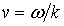and the absorption coefficient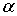:, (1)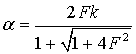, (2)

where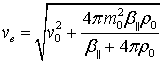, (3)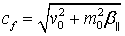, (4), (5)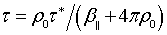,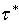– relaxation time of the magnetic field to its equilibrium value in a magnetic fluid,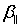– magnetoelastic parameter,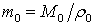– unperturbed specific magnetization value,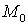– unperturbed magnetization value,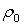unperturbed magnetic fluid density,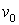– sound velocity in magnetic fluid without exposure of external magnetic field,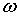– sound cyclic frequency,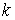– sound wave number.

The unitless parameter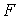(5) depends on theand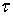resonantly, so in the cases of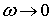and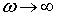,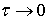and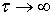it tends to zero, and when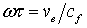in the maximum it takes the form :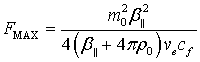. (6)

In the case of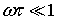the sound velocity (1)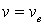equals the sound wave velocity (3) in an ideal magnetic fluid with equilibrium magnetization in the propagation parallel to the magnetic field . In the case of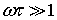the sound velocity (1)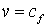equals the fast magnetoacoustic wave velocity (4) in an ideal magnetic fluid with frozen-in magnetization under parallel propagation to the magnetic field [2, 12].

For the magnetic nanofluid EMG – 605 [2, 7, 8, 12]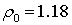g/cm3 and the parameter maximum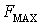(6) numerical value approximately equals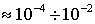. Here, inequality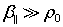was used, which is confirmed by comparing the theoretical calculations with experimental data [2, 11, 12], so in linear approximation the sound  absorption coefficient (2) is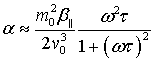. (7)

With constant cyclic frequencyinvestigating the dependence ​​onfor (7), so with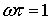the maximum value of the absorption coefficient is approximately equal to :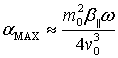. (8)

In the case ofthe absorption coefficient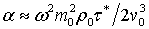is a small quantity relative to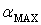, i. e.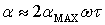.

In the case ofthe absorption coefficient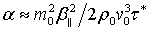is also a small quantity relative to, i. e.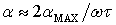.

In  it was shown for the magnetic nanofluid EMG – 605  that in the interval for the magnetic field of 30 kA/m to 120 kA/m the theoretical dependence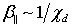was confirmed with good accuracy. So this theory [9, 10] can be used to explain ultrasonic absorption peaks in . In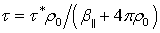increased approximately 100 times, and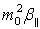increased approximately 6 times on the mentioned above interval. In  analytical functions were used to approximate the experimental data on the change in the ultrasound velocity in the magnetic nanofluids with dependence on the exposure time of the static magnetic field. It was shown that relaxation timetends to zero then the exposure time is small, and the relaxation product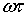can be approximated by this exponential dependence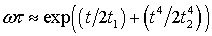then the exposure time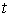is sufficiently large, where the characteristic times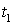and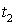are approximately about hour . So, according to technique of mathematical estimation , this relaxation timeis a fast function in formula (7), and the compositionis a slow function then the factor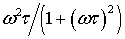depends on the relaxation timeresonantly. In experiments  the cyclic frequencyis constant and the magnetic field is increased slowly from time to time. Therefore in Fig. the peak theoretical values (circles) were calculated by the formula (8). The sound velocity in magnetic fluid without exposure of external magnetic field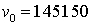cm/s, the numerical values of magnetoelastic parameterand specific magnetization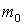were taken from . In Fig. 1 the experimental data (triangles) are the numerical values of ultrasonic absorption maxima from Fig. 2 in  for sweep times 20, 40, 60, 120 and 200 minutes. The theoretical values are a little more than the experimental data. It is good, because the theoretical calculations were made under condition. Moreover, for 90 kA/m theoretical value (8)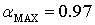cm-1 is larger than the maximum experimental value, which is approximately equal to 0.8 cm-1 in Fig. 2 of .

In Fig. 1 experimental datum is very close to theoretical value under magnetic field 39 kA/m. So, using the conditionand ultrasonic frequency 3.6 MHz , relaxation time is approximately equal to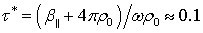s. Parameter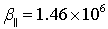g/cm3 was taken from . In  also water-based magnetic nanofluid W – 40 was considered, but with other concentration of magnetic nanoparticles and magnetic field was static with value 100 mT. However, according to the formula (20) in  relaxation time is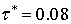s at the maximum absorption coefficient, which is very close to mentioned above relaxation time.

In experiment  the absorption peak is smooth in magnetic nanofluid APG-832 on the exposure of the static magnetic field. Also in theoretical calculations  the absorption peaks are smooth for magnetic nanofluids W – 40 and HC-50 on the exposure of the static magnetic field. In experiments  the absorption peaks are sharp for the magnetic nanofluid EMG – 605, which may be due to the fact that the magnetic field increased on the time, so the relaxation timecan increase faster than on the exposure of the static magnetic field.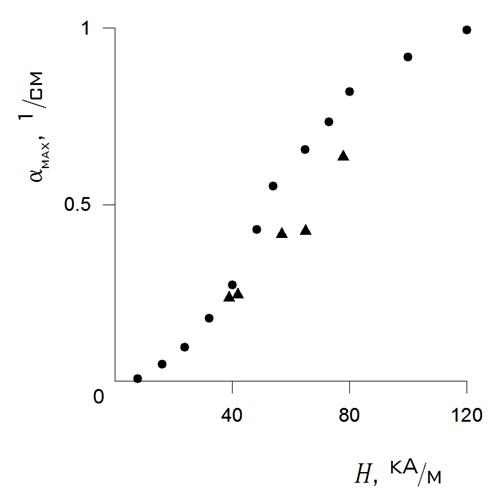Fig. 1. The maxima of ultrasonic absorption coefficient: circles – the theoretical values by the formula (8) and triangles the experimental data , with dependence on magnetic field strength.

3. Conclusion

The magnetic nanofluid is a complex system, so in it the magneto-mechanical properties may depend on the conditions of the experiment [2, 7, 8]. Big peaks of ultrasonic absorption coefficient were observed under the rate of magnetic field in experiments , which is connected with irreversible process of formation of chain-like clusters. In this article these peaks can be explained by the fact that there is a relaxation process of magnetic field to its equilibrium value in magnetic nanofluid [9, 10]. Thus, formation of chain-like clusters is connected with a relaxation process of magnetic field to its equilibrium value.

References

 R. E. Rosensweig. Ferrohydrodynamics, Cambridge Univ. Press, New York, 1985. 368p.

 A. Józefczak, T. Hornowski, V. Závišová, A. Skumiel, M. Kubovčíková, M. Timko. Acoustic Wave in a Suspension of Magnetic Nanoparticle with Sodium Oleate Coating // J. Nanopart. Res. 2014, Vol. 16, p. 2271. DOI:  10.1007/s11051-014-2271-z

 C.G. Montgomery. The Magnetization of Colloidal Suspensions // Phys. Rev. 1931. Vol. 38. No. 9. p. 1782.

 W.C. Elmore. Ferromagnetic Colloid for Studying Magnetic Structures // Phys. Rev. 1938. Vol. 54. No. 4. p. 309-310.

 W.C. Elmore. The Magnetization of Ferromagnetic Colloids // Phys. Rev. 1938. Vol. 54, No. 12. p. 1092-1095.

 S.S. Papell. Low Viscosity Magnetic Fluid Obtained by the Colloidal Suspension of Magnetic Particles, US Patent No 3215572, 1965.

 A. Józefczak, M. Łabowski, A. Skumiel. Hysteresis of Changes of Ultrasonic Wave Absorption Coefficient in a Magnetic Fluid Caused by the Magnetic Field // J. Magn. Magn. Mat. 2002, Vol. 252, p. 356359.

 T. Hornowski. Ultrasonic Properties of EMG-605 Magnetic Liquid // Proc. SPIE. 2005. Vol. 5828. p. 205-212. http://dx.doi.org/10.1117/12.612810

 V.V. Sokolov, V.V. Tolmachev. Propagation of Shear Waves in a Magnetic Liquid with Frozen-In Magnetization // Tech. Phys. Lett. 1997. Vol. 23, No. 1 p.5-6. DOI: 10.1134/1.1261628

 B.U. Felderhof, V.V. Sokolov, P.A. Eminov. Ferrofluid Dynamics, Magnetic Relaxation and Irreversible Thermodynamics // J. Chem. Phys. 2010. Vol. 132. No. 18. p. 184907.

 I.E. Ovchinnikov. Effect of a Static Magnetic Field on the Ultrasonic Propagation in Magnetic Fluids // J. Radio Electronics. 2013. N1. URL:   [in Russian with abstract in English]

 I.E. Ovchinnikov, V.V. Sokolov. Waves in Magnetic Fluids with Equilibrium

and Frozen-In Magnetizations // Acoust. Phys., 2013. Vol. 59, No. 1, p. 51-

55. DOI:  10.1134/S1063771012060115

 I.E. Ovchinnikov. Effect of a Magnetic Field Relaxation on the Splash of Ultrasonic Absorption Coefficient in Magnetic Nanofluid EMG – 605 // J. Radio Electronics.  2014. N1. URL:  http://jre.cplire.ru/jre/jan14/17/text.pdf [in Russian with abstract in English]

 A. B. Migdal. Qualitative Methods in Quantum Theory, W.A. Benjamin, Inc. London. 1977. 437p.

 K. Charaziak, M. Marecki, T. Hornowski, A. Józefczak, A. Skumiel. Resonance Attenuation of Ultrasonic Waves in a High Viscosity Magnetic Liquid // Arch. Acoust. 2008, Vol. 33 (4, Suppl.), p. 105–110.